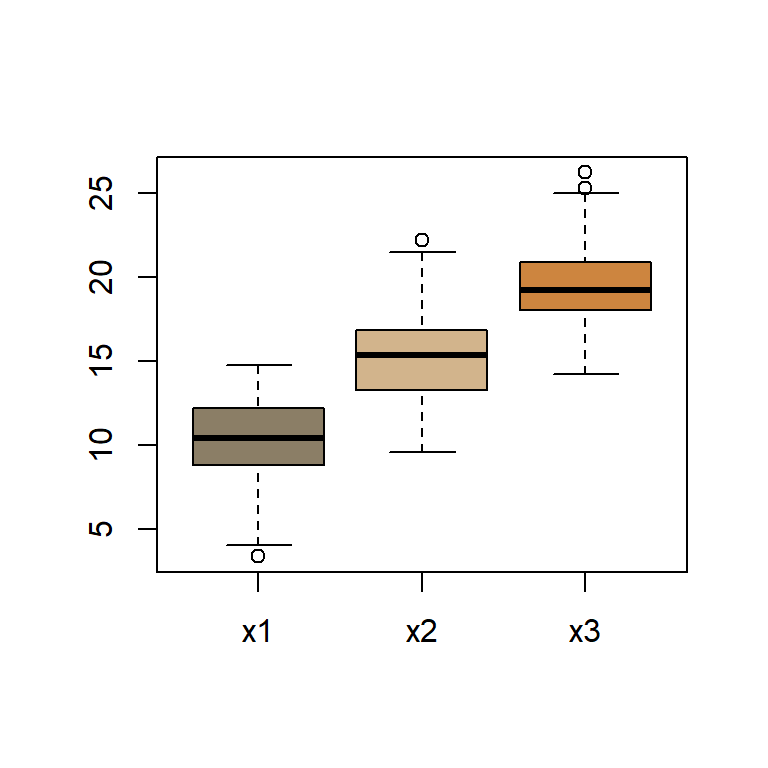### INTERPRETATION OF THE RESULTS OF AN ANOVA TEST IN R:

An “Analysis of Variance” (ANOVA) tests three or more groups for mean differences based on a continuous (i.e. scale or interval) response variable (a.k.a. dependent variable). The term “factor” refers to the variable that distinguishes this group membership. Race, level of education, and treatment condition are examples of factors.

There are two main types of ANOVA: (1) “one-way” ANOVA compares levels (i.e. groups) of a single factor based on single continuous response variable (e.g. comparing test score by ‘level of education’) and (2) a “two-way” ANOVA compares levels of two or more factors for mean differences on a single continuous response variable(e.g. comparing test score by both ‘level of education’ and ‘zodiac sign’). In practice, you will see one-way ANOVAs more often and when the term ANOVA is generically used, it often refers to a one-way ANOVA. Henceforth in this blog entry, I use the term ANOVA to refer to the one-way flavor.

The essential computation is the F-test:

$F=\frac{\text{variance between treatments}}{\text{variance within treatments}}=\large\frac{\frac{\text{Sum Sqs}_{\text{treat'ts}}}{\text{no. treat'ts}-1}}{\frac{\text{Sum Sqs}_{\text{err's}}}{\text{no. cases}-\text{no. treat'ts}}}$,

Let’s create three normally distributed samples with the same variance, but different means, and run an anova in R:

set.seed(1); n = 50; x1 = rnorm(n, 10, 3); x2 = rnorm(n, 15, 3); x3 = rnorm(n, 20, 3)
dataframe = data.frame(x1,x2,x3)
boxplot(dataframe, col=c("wheat4", "tan", "tan3"))Now let’s calculate the anova manually:

datamatrix = as.matrix(dataframe)
(global.mean = mean(datamatrix))                                    # The overall mean
##  15.06529
(group.means = apply(datamatrix, 2, mean))                          # The respective means for each group
##       x1       x2       x3
## 10.30134 15.35198 19.54254
(SST = sum((datamatrix - global.mean)^2))                           # Sum squares total
##  3216.922
(SSBetween = sum(nrow(datamatrix) * (group.means-global.mean)^2))   # Between groups
##  2141.158
(SSWithin = sum(scale(datamatrix, center = T, scale = F)^2))        # Sum Squares Residuals
##  1075.764
(MeanSqBetween = SSBetween / (ncol(datamatrix) - 1))                # Between/df(groups - 1) [3 - 1]
##  1070.579
(MeanSqWithin = SSWithin / (length(datamatrix) - ncol(datamatrix))) # Within/df(subjects - no.groups) [150-3]
##  7.318123
(F_value = MeanSqBetween / MeanSqWithin)                            # F value
##  146.2915

Compare to the R function. First let’s ready up the data:

datalong = stack(dataframe)
fit = aov(values ~ ind, datalong); summary(fit)
##              Df Sum Sq Mean Sq F value Pr(>F)
## ind           2   2141  1070.6   146.3 <2e-16 ***
## Residuals   147   1076     7.3
## ---
## Signif. codes:  0 '***' 0.001 '**' 0.01 '*' 0.05 '.' 0.1 ' ' 1

Alternatively we can resort to the OLS function:

OLS = lm(values ~ ind, datalong); summary(OLS); anova(OLS)
##
## Call:
## lm(formula = values ~ ind, data = datalong)
##
## Residuals:
##     Min      1Q  Median      3Q     Max
## -6.9454 -1.5772 -0.1468  1.5970  6.8529
##
## Coefficients:
##             Estimate Std. Error t value Pr(>|t|)
## (Intercept)  10.3013     0.3826  26.926   <2e-16 ***
## indx2         5.0506     0.5410   9.335   <2e-16 ***
## indx3         9.2412     0.5410  17.080   <2e-16 ***
## ---
## Signif. codes:  0 '***' 0.001 '**' 0.01 '*' 0.05 '.' 0.1 ' ' 1
##
## Residual standard error: 2.705 on 147 degrees of freedom
## Multiple R-squared:  0.6656, Adjusted R-squared:  0.661
## F-statistic: 146.3 on 2 and 147 DF,  p-value: < 2.2e-16
## Analysis of Variance Table
##
## Response: values
##            Df Sum Sq Mean Sq F value    Pr(>F)
## ind         2 2141.2 1070.58  146.29 < 2.2e-16 ***
## Residuals 147 1075.8    7.32
## ---
## Signif. codes:  0 '***' 0.001 '**' 0.01 '*' 0.05 '.' 0.1 ' ' 1

####PAIRWISE COMPARISONS:

Additional analyses are required after an omnibus test. The function pairwise.t.test() computes the pair-wise comparisons between group means with corrections for multiple testing:

pairwise.t.test(reponse, factor, p.adjust = method, alternative = c("two.sided", "less", "greater"))

Response is a vector of observations (the response variable), factor a list of factors and p.adjust is the correction method (e.g., “Bonferroni”).

options(scipen=999)
pairwise.t.test(datalong$values, datalong$ind, p.adjust="bonferroni")
##
##  Pairwise comparisons using t tests with pooled SD
##
## data:  datalong$values and datalong$ind
##
##    x1                   x2
## x2 0.00000000000000044  -
## x3 < 0.0000000000000002 0.00000000000428679
##
## P value adjustment method: bonferroni

All the pairwise comparisons are statistically significant.

Alternatively, a Tukey test can be undertaken:

TukeyHSD(fit)
##   Tukey multiple comparisons of means
##     95% family-wise confidence level
##
## Fit: aov(formula = values ~ ind, data = datalong)
##
## \$ind
##           diff      lwr       upr p adj
## x2-x1 5.050635 3.769616  6.331653     0
## x3-x1 9.241199 7.960181 10.522217     0
## x3-x2 4.190564 2.909546  5.471583     0

The differences pairwise and the p-values are shown.